#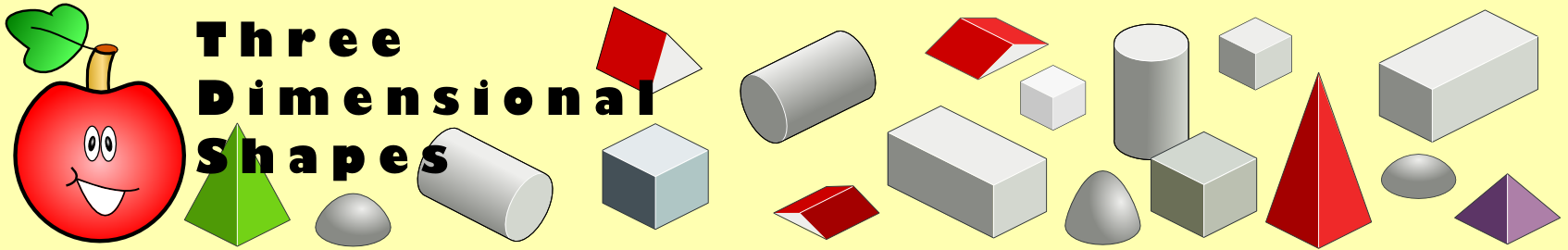There are 366 different Starters of The Day, many to choose from. You will find below some starters on the topic of Shape (3D). A lesson starter does not have to be on the same topic as the main part of the lesson or the topic of the previous lesson. It is often very useful to revise or explore other concepts by using a starter based on a totally different area of Mathematics.

Main Page

### Shape (3D) Starters: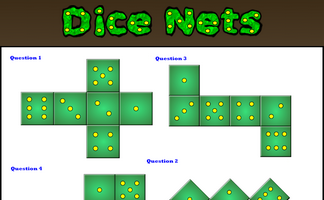Determine whether the given nets would fold to produce a dice.A dice is reflected in two mirrors. What numbers can be seen?Find the number of faces, edges and vertices on some familiar objects.How many triangles are there on the surface of a regular icosahedron.

## Exercises#### 3D Trigonometry Presentation

A slide presentation (a poem) introducing using trigonometry (including Pythagoras' Theorem) to find lengths and angles on three dimensional shapes.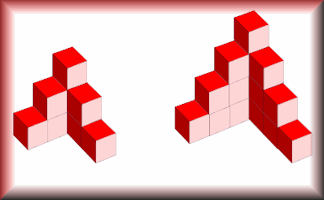#### Cube Construction

This is a simple interactive that does nothing more than allow you to create 3D drawings of models made with cubes.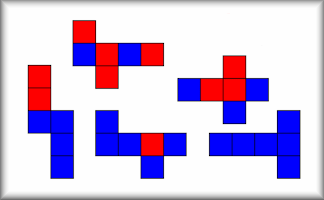#### Cubical Net Challenge

Find all the ways of painting the faces of cubes using only two colours.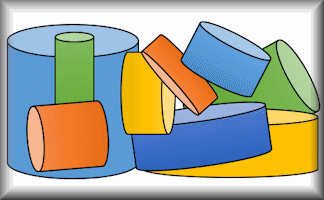#### Cylinders

Apply formulae for the volumes and surface areas of cylinders to answer a wide variety of questions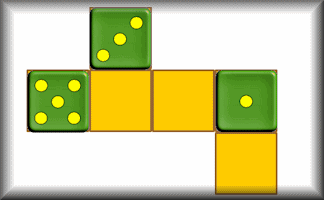#### Dice Net Challenge

Drag the numbers onto the net so that when it is folded to form a cube numbers on opposite faces add up to prime numbers.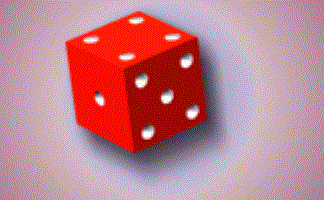#### Faces, Edges and Vertices

Calculate the number of faces, edges and vertices on 3D Shapes.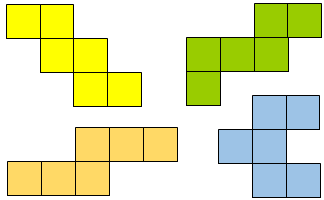#### Net or Not

Drag the nets into the corresponding panels to show whether they would fold to form a cube.#### Plans and Elevations

Interpret plans and elevations of three dimensional shapes.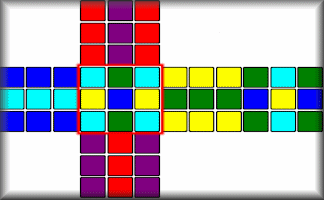#### Puzzle Cube Net

A jumbled moving-block puzzle cube is shown as a net. Can you solve it?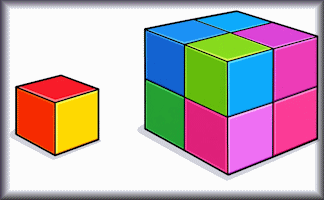#### Similar Shapes

Questions about the scale factors of lengths, areas and volumes of similar shapes.#### Surface Area

Work out the surface areas of the given solid shapes.#### The Great Dodecahedron

Pupils are not allowed to use their hands to point but must describe fully any shapes they can see in this picture.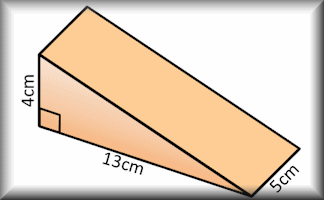#### Volume

Use formulae to solve problems involving the volumes of cuboids, cones, pyramids, prisms and composite solids.#### Yes No Questions

A game to determine the mathematical item by asking questions that can only be answered yes or no.

### Search

The activity you are looking for may have been classified in a different way from the way you were expecting. You can search the whole of Transum Maths by using the box below.

Have today's Starter of the Day as your default homepage. Copy the URL below then select
Tools > Internet Options (Internet Explorer) then paste the URL into the homepage field.

Set as your homepage (if you are using Internet Explorer)

Do you have any comments? It is always useful to receive feedback and helps make this free resource even more useful for those learning Mathematics anywhere in the world. Click here to enter your comments.For All: